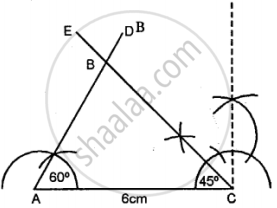# Construct Traingle Abc, When : Ac = 6 Cm, ∠A = 60“ and ∠C = 45°. Measure Ab and Bc. - Mathematics

Diagram
Sum

Construct traingle ABC, when : AC = 6 cm, ∠A = 60“ and ∠C = 45°. Measure AB and BC.

#### Solution

Steps of Construction:

(1) Draw a line segment AC = 6 cm.(2) At A construct an angle ∠A = 60°.

(3) At C construct an angle ∠C = 45°.

(4) AD and CE intersect each other at B.

(5) ∴ ∆ABC is the required triangle.

(6) On measuring AB = 4-4 cm, BC = 5.4 cm.

Concept: Classification of Triangles (On the Basis of Sides, and of Angles)
Is there an error in this question or solution?

#### APPEARS IN

Selina Class 6 Mathematics
Chapter 26 Triangles (Including Types, Properties and Constructions)
Exercise 26 (B) | Q 7